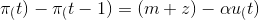### Create an Account

Already have account?

### Forgot Your Password ?

Home / Questions / If the Phillips curve equation is represented by equation: (t) – t – 1) = (m + 2) – aut) a...

# If the Phillips curve equation is represented by equation: (t) – t – 1) = (m + 2) – aut) and the change in the inflation rate equals 20 - 2u, 1) what is the natural rate of unemployment in the econom

If the Phillips curve equation is represented by equation:and the change in the inflation rate equals 20 - 2u,

1) what is the natural rate of unemployment in the economy?

a. 10%.

b. 20%.

c. 6.5%.

d. 5%.

e. none of the above

2) which of the following is most likely to occur if the actual unemployment in any period is equal to 6%?

a. the rate of inflation will tend to increase

b. the rate of inflation will be constant

c. the rate of inflation will tend to decrease

d. none of the above

Apr 16 2021 View more View Less

#### Answer (Solved)Subscribe To Get Solution